## 一、 numpy 数组

1.创建numpy ndarry对象

``````import numpy as np

arr = np.array([1, 2, 3, 4, 5])

print(arr)

print(type(arr))
``````
• 1
• 2
• 3
• 4
• 5
• 6
• 7

2.数组中的维

（1）0维度数组，也就是标量

``````import numpy as np

arr = np.array(61)

print(arr)

``````
• 1
• 2
• 3
• 4
• 5
• 6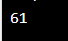（2）1维数组

``````import numpy as np

arr = np.array((1, 2, 3, 4, 5))

print(arr)
``````
• 1
• 2
• 3
• 4
• 5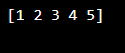（3）2维数组

``````import numpy as np

arr = np.array([[1, 2, 3], [4, 5, 6]])

print(arr)
``````
• 1
• 2
• 3
• 4
• 5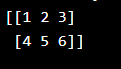（4）3维数组

``````import numpy as np

arr = np.array([[[1, 2, 3], [4, 5, 6]], [[1, 2, 3], [4, 5, 6]]])

print(arr)
``````
• 1
• 2
• 3
• 4
• 5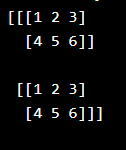（5）n维数组

``````import numpy as np

arr = np.array([1, 2, 3, 4], ndmin=5)

print(arr)
print('number of dimensions :', arr.ndim)

``````
• 1
• 2
• 3
• 4
• 5
• 6
• 7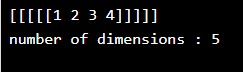3.数组索引
（1）访问数组元素

NumPy 数组中的索引以 0 开头，这意味着第一个元素的索引为 0，第二个元素的索引为 1，以此类推。

``````import numpy as np

arr = np.array([1, 2, 3, 4])

print(arr)
``````
• 1
• 2
• 3
• 4
• 5

``````import numpy as np

arr = np.array([[1,2,3,4,5], [6,7,8,9,10]])

print('2nd element on 1st dim: ', arr[0, 1])
``````
• 1
• 2
• 3
• 4
• 5

``````import numpy as np

arr = np.array([[[1, 2, 3], [4, 5, 6]], [[7, 8, 9], [10, 11, 12]]])

print(arr[0, 1, 2])
``````
• 1
• 2
• 3
• 4
• 5

（2）负索引

``````import numpy as np

arr = np.array([[1,2,3,4,5], [6,7,8,9,10]])

print('Last element from 2nd dim: ', arr[1, -1])
``````
• 1
• 2
• 3
• 4
• 5

## 二、 Numpy数组裁切

1.裁切数组
python 中裁切的意思是将元素从一个给定的索引带到另一个给定的索引。

2.负裁切

``````import numpy as np

arr = np.array([1, 2, 3, 4, 5, 6, 7])

print(arr[-3:-1])
``````
• 1
• 2
• 3
• 4
• 5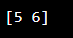## 三、Numpy 数据类型

1.Python 中的数据类型

strings – 用于表示文本数据，文本用引号引起来。例如 “ABCD”。
integer – 用于表示整数。例如 -1, -2, -3。
float – 用于表示实数。例如 1.2, 42.42。
boolean – 用于表示 True 或 False。
complex – 用于表示复平面中的数字。例如 1.0 + 2.0j，1.5 + 2.5j。
2.常见错误

ValueError：在 Python 中，如果传递给函数的参数的类型是非预期或错误的，则会引发 ValueError。
3.转换已有数组的数据类型

astype() 函数创建数组的副本，并允许您将数据类型指定为参数。

1.副本和视图之间的区别

2.检查数组是否拥有数据

## 五、NumPy 数组形状

1.数组的形状

2.获取数组的形状
NumPy 数组有一个名为 shape 的属性，该属性返回一个元组，每个索引具有相应元素的数量。

``````import numpy as np

arr = np.array([[1, 2, 3, 4], [5, 6, 7, 8]])

print(arr.shape)
``````
• 1
• 2
• 3
• 4
• 5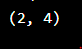3.元组的形状代表什么？

## 六、数组重塑

1.数组重塑

2.从 1-D 重塑为 2-D

``````import numpy as np

arr = np.array([1, 2, 3, 4, 5, 6, 7, 8, 9, 10, 11, 12])

newarr = arr.reshape(4, 3)

print(newarr)
``````
• 1
• 2
• 3
• 4
• 5
• 6
• 7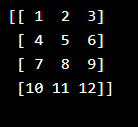3.从 1-D 重塑为 3-D

``````import numpy as np

arr = np.array([1, 2, 3, 4, 5, 6, 7, 8, 9, 10, 11, 12])

newarr = arr.reshape(2, 3, 2)

print(newarr)
``````
• 1
• 2
• 3
• 4
• 5
• 6
• 7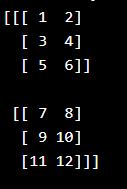4.我们可以重塑成任何形状吗？

5.返回副本还是视图？

``````import numpy as np

arr = np.array([1, 2, 3, 4, 5, 6, 7, 8])

print(arr.reshape(2, 4).base)
``````
• 1
• 2
• 3
• 4
• 5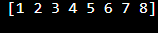6.未知的维

``````import numpy as np
arr = np.array([1, 2, 3, 4, 5, 6, 7, 8])
newarr = arr.reshape(2, 2, -1)
print(newarr)
``````
• 1
• 2
• 3
• 4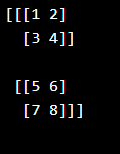7.展平数组

``````import numpy as np

arr = np.array([[1, 2, 3], [4, 5, 6]])

newarr = arr.reshape(-1)

print(newarr)
``````
• 1
• 2
• 3
• 4
• 5
• 6
• 7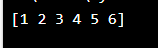## 七、数组迭代

1.数组迭代

2.如果我们对 1-D 数组进行迭代，它将逐一遍历每个元素。

``````import numpy as np

arr = np.array([1, 2, 3])

for x in arr:
print(x)
``````
• 1
• 2
• 3
• 4
• 5
• 6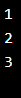3.迭代 2-D 数组

``````import numpy as np

arr = np.array([[1, 2, 3], [4, 5, 6]])

for x in arr:
print(x)
``````
• 1
• 2
• 3
• 4
• 5
• 6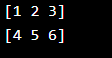4.如果我们迭代一个 n-D 数组，它将逐一遍历第 n-1 维。

``````import numpy as np

arr = np.array([[1, 2, 3], [4, 5, 6]])

for x in arr:
for y in x:
print(y)
``````
• 1
• 2
• 3
• 4
• 5
• 6
• 7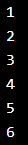5.迭代 3-D 数组

``````import numpy as np

arr = np.array([[[1, 2, 3], [4, 5, 6]], [[7, 8, 9], [10, 11, 12]]])

for x in arr:
print(x)
``````
• 1
• 2
• 3
• 4
• 5
• 6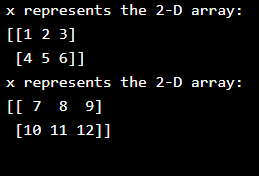6.要返回实际值、标量，我们必须迭代每个维中的数组

``````import numpy as np

arr = np.array([[[1, 2, 3], [4, 5, 6]], [[7, 8, 9], [10, 11, 12]]])

for x in arr:
for y in x:
for z in y:
print(z)
``````
• 1
• 2
• 3
• 4
• 5
• 6
• 7
• 8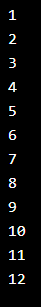7.使用 nditer() 迭代数组

``````import numpy as np

arr = np.array([[[1, 2], [3, 4]], [[5, 6], [7, 8]]])

for x in np.nditer(arr):
print(x)
``````
• 1
• 2
• 3
• 4
• 5
• 6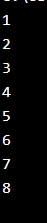8.迭代不同数据类型的数组

NumPy 不会就地更改元素的数据类型（元素位于数组中），因此它需要一些其他空间来执行此操作，该额外空间称为 buffer，为了在 nditer() 中启用它，我们传参 flags=[‘buffered’]。

``````import numpy as np

arr = np.array([1, 2, 3])

for x in np.nditer(arr, flags=['buffered'], op_dtypes=['S']):
print(x)
``````
• 1
• 2
• 3
• 4
• 5
• 6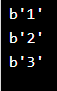9.以不同的步长迭代

``````import numpy as np

arr = np.array([[1, 2, 3, 4], [5, 6, 7, 8]])

for x in np.nditer(arr[:, ::2]):
print(x)
``````
• 1
• 2
• 3
• 4
• 5
• 6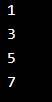10.使用 ndenumerate() 进行枚举迭代

``````import numpy as np

arr = np.array([1, 2, 3])

for idx, x in np.ndenumerate(arr):
print(idx, x)
``````
• 1
• 2
• 3
• 4
• 5
• 6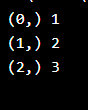``````import numpy as np

arr = np.array([[1, 2, 3, 4], [5, 6, 7, 8]])

for idx, x in np.ndenumerate(arr):
print(idx, x)
``````
• 1
• 2
• 3
• 4
• 5
• 6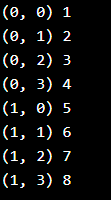## 八、数组连接

1.连接 NumPy 数组

2.实例

``````import numpy as np

arr1 = np.array([1, 2, 3])

arr2 = np.array([4, 5, 6])

arr = np.concatenate((arr1, arr2))

print(arr)
``````
• 1
• 2
• 3
• 4
• 5
• 6
• 7
• 8
• 9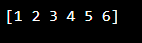3.实例

``````import numpy as np

arr1 = np.array([[1, 2], [3, 4]])

arr2 = np.array([[5, 6], [7, 8]])

arr = np.concatenate((arr1, arr2), axis=1)

print(arr)
``````
• 1
• 2
• 3
• 4
• 5
• 6
• 7
• 8
• 94.使用堆栈函数连接数组

``````import numpy as np

arr1 = np.array([1, 2, 3])

arr2 = np.array([4, 5, 6])

arr = np.stack((arr1, arr2), axis=1)

print(arr)
``````
• 1
• 2
• 3
• 4
• 5
• 6
• 7
• 8
• 9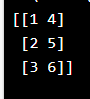5.沿行堆叠
NumPy 提供了一个辅助函数：hstack() 沿行堆叠。

``````import numpy as np

arr1 = np.array([1, 2, 3])

arr2 = np.array([4, 5, 6])

arr = np.hstack((arr1, arr2))

print(arr)
``````
• 1
• 2
• 3
• 4
• 5
• 6
• 7
• 8
• 9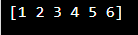6.沿列堆叠
NumPy 提供了一个辅助函数：vstack() 沿列堆叠。

``````import numpy as np

arr1 = np.array([1, 2, 3])

arr2 = np.array([4, 5, 6])

arr = np.vstack((arr1, arr2))

print(arr)
``````
• 1
• 2
• 3
• 4
• 5
• 6
• 7
• 8
• 97.沿高度堆叠（深度）
NumPy 提供了一个辅助函数：dstack() 沿高度堆叠，该高度与深度相同。

``````import numpy as np

arr1 = np.array([1, 2, 3])

arr2 = np.array([4, 5, 6])

arr = np.dstack((arr1, arr2))

print(arr)
``````
• 1
• 2
• 3
• 4
• 5
• 6
• 7
• 8
• 9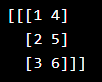## 九、数组拆分

1.拆分 NumPy 数组

2.实例

``````import numpy as np

arr = np.array([1, 2, 3, 4, 5, 6])

newarr = np.array_split(arr, 3)

print(newarr)
``````
• 1
• 2
• 3
• 4
• 5
• 6
• 7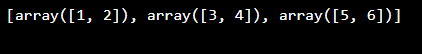3.如果数组中的元素少于要求的数量，它将从末尾进行相应调整。

``````import numpy as np

arr = np.array([1, 2, 3, 4, 5, 6])

newarr = np.array_split(arr, 4)

print(newarr)
``````
• 1
• 2
• 3
• 4
• 5
• 6
• 7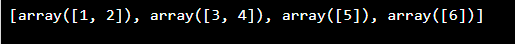4.拆分为数组
array_split() 方法的返回值是一个包含每个分割的数组。

``````import numpy as np

arr = np.array([1, 2, 3, 4, 5, 6])

newarr = np.array_split(arr, 3)

print(newarr)
print(newarr)
print(newarr)
``````
• 1
• 2
• 3
• 4
• 5
• 6
• 7
• 8
• 9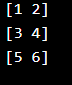5.分割二维数组

``````import numpy as np

arr = np.array([[1, 2], [3, 4], [5, 6], [7, 8], [9, 10], [11, 12]])

newarr = np.array_split(arr, 3)

print(newarr)
``````
• 1
• 2
• 3
• 4
• 5
• 6
• 7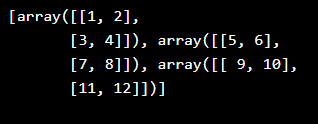## 十、数组搜索

1.搜索数组

``````import numpy as np

arr = np.array([1, 2, 3, 4, 5, 4, 4])

x = np.where(arr == 4)

print(x)
``````
• 1
• 2
• 3
• 4
• 5
• 6
• 7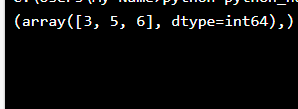Nupmy 随机。